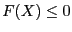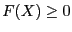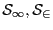Next: The 3B method Up: Mathematical background Previous: An alternative: the single   Contents

Solutions and Distinct solutions

An interval will be considered as a solution for a function of the system in the following cases:

• for equations the maximal diameter of the intervals is less than a given threshold epsilon and the corresponding interval evaluation of the function contains 0 or the corresponding interval evaluation of the function has a diameter less than a given threshold epsilonf and the interval contains 0
• for inequalities: the upper bound of the interval evaluation of the function is negative or the maximal diameter of the intervals is less than a given threshold epsilon and the corresponding interval evaluation of the function has at least a negative lower bound or the corresponding interval evaluation of the function has a diameter less than a given threshold epsilonf and the interval contains 0
• for inequalities: the lower bound of the interval evaluation of the function is positive or the maximal diameter of the intervals is less than a given threshold epsilon and the corresponding interval evaluation of the function has at least a positive upper bound or the corresponding interval evaluation of the function has a diameter less than a given threshold epsilonf and the interval contains 0
A solution of the system is defined as a box such that the above conditions hold for each function of the system. Note that for systems having interval coefficients (which are indicated by setting the flag ALIAS_Func_Has_Interval to 1) a solution of a system will be obtained only if the inequalities are strictly verified.

Assume that two solutionshave been found with the algorithm. We will first consider the case where we have to solve a system ofequations inunknowns, possible with additional inequality constraints. First we will check with the Miranda theorem (see section 3.1.5) ifinclude one (or more) solution(s). If both solutions are Miranda, then they will kept as solutions. If one of them is Miranda and other one is not Miranda we will consider the distance between the mid-point of: if this distance is lower than a given threshold we will keep as solution only the Miranda's one. If none ofis Miranda we keep these solutions, provided that their distance is greater than the threshold. Note that in that case these solutions may disappear if a Miranda solution is found later on such that the distance between these solutions and the Miranda's one is lower than the threshold.

In the other case the solution will be ranked according the chosen order and if a solution is at a distance from a solution with a better ranking lower than the threshold, then this solution will be discarded.Next: The 3B method Up: Mathematical background Previous: An alternative: the single   Contents
Jean-Pierre Merlet 2012-12-20